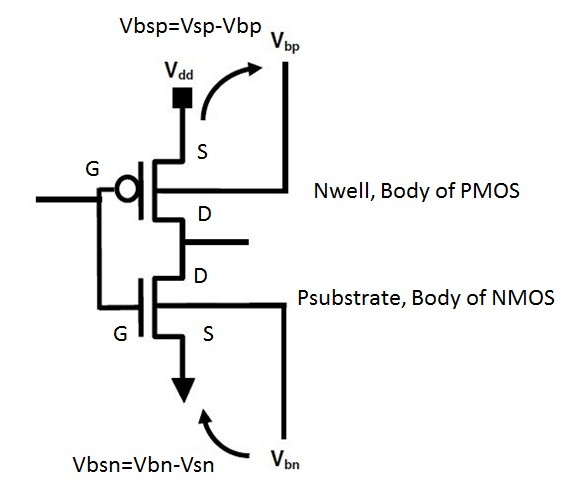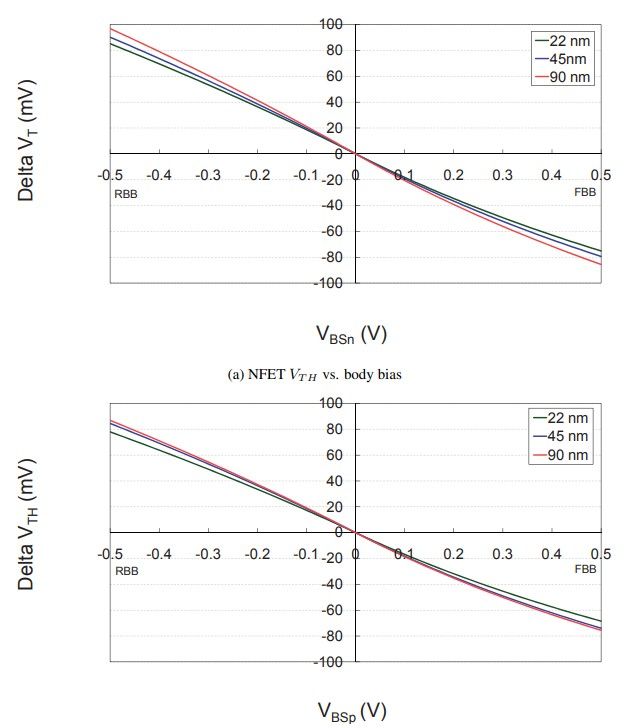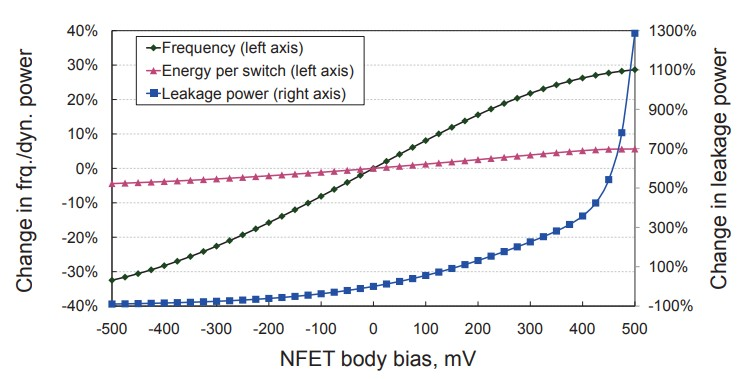# Body Biasing and PFET Nwell Connection

Make it to the Right and Larger Audience

Blog

# Body Biasing and PFET Nwell Connection

What is body and substrate biasing? How to connect PFET nwell? It is supposed to be a simple topic until I google it. Lots of talks but confusing or missing the point. Finally I run into a blog from Semiengineering, Substrate Biasing , which gives a excellent introduction about this topic.

“Substrate biasing in PMOS biases the body of the transistor to a voltage higher than Vdd; in NMOS, to a voltage lower than Vss.
Since leakage currents are a function of device Vth, substrate biasing-also known as back biasing-can reduce leakage power. With this advanced technique, the substrate or the appropriate well is biased to raise the transistor thresholds, thereby reducing leakage. In PMOS, the body of transistor is biased to a voltage higher than Vdd. In NMOS, the body of transistor is biased to a voltage lower than Vss.”Here we go:

Vgn, Vsn, Vdn, Vbn are voltage of NMOS gate, source, drain, and body (Psubstrate). Vgp, Vsp, Vdp, Vbp are voltage of PMOS gate, source, drain and body (Nwell).

Normally Vsn is connected to ground so Vsn=0 and Vsp is connected to digital supply Vdd so Vsp=Vdd.

If NMOS is not body biased, Vbn is connected to Vsn so Vbn=Vsn=0.

If PMOS is not body biased, Vbp is connected to Vsp so Vbp=Vsp=Vdd.

RBB is reverse body bias or back biasing. In this case, for NMOS, Vbn<Vsn so Vbsn=Vbn-Vsn is a negative number. For PMOS, Vbp>Vsp so Vbsp=Vsp-Vbp is also a negative number.

FBB is forward body bias. In this case, for NMOS, Vbn>Vsn so Vbsn=Vbn-Vsn is a positive number. For PMOS, Vbp<Vsp so Vbsp=Vsp-Vbp is also a positive number.

RBB increases Vth which means lower leakage but slower speed. Think about HVT (high Vth) standard cell.

FBB decreases Vth which means higher leakage but faster speed. Think about LVT (low Vth) standard cell.

Now let’s introduce a little bit math from  Reducing Power using Body Biasing in Microprocessors With Dynamic Voltage/Frequency Scaling.As can be seen, if Vbs<0 in RBB case, Vth increases. Makes sense. Below diagram illustrates this relationship of Vbs vs Vth for both NMOS and PMOS.Relationship of NMOS Vbsn vs leakage and speed is shown in the following figure. PMOS follows the similar pattern.Note:

1. In RBB case, how high Vbp can go and how low Vbn can go is process node dependent. There is a limit and beyond that standard cell does not work.
2. In FBB, there is a risk of latchup since PMOS Nwell, Vbp, is not the highest potential.Author brief is empty
Groups:

Tags: